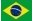Dimensions of mass     M = L³ / T²Given the principle that the same physical quantity will always have the same dimensions (a surface, for example, will always be defined by the square of its length, L² ; linear speed will always be defined as the relationship between distance and time, L / T), the force resulting from the acceleration of mass and the force resulting from the attraction between masses should always have the same dimensions. According to the equivalence principle both forces are considered identical. It follows that the Newton constant will have no dimension and that mass will not be a fundamental quantity and will have as its dimensions L³/T². The unit of mass will become m³ / s² and 1kg of mass will be 8,3868 10^-10  m³ / s² (see Appendix 2). Since the unit of mass is (metre)³ / (second)² , or ( m³ / s² ), the Newton constant will be  1/4 p In Lorentz–Heaviside units, also called rationalized, the Coulomb constant is  also  1/4 p ( pt.wikipedia ) The electric charge will have the same dimension as the mass, but will be complex: i L³/T². Many of the physical quantities like force, movement, energy, etc. will depend exclusively on distance (L) and time (T). Electrical conductivity will be in the same dimension as speed and capacity as distance. The electrical quantities (charge, potential, intensity, electric field) will become unmeasurable complex quantities, contrary to mechanical quantities, that will be real and directly measurable. To measure a complex electrical quantity (charge, potential, intensity, electric field) it will be necessary to use electricity in order to obtain a real, measurable quantity (force or displacement, for example). A certain electric charge will give out measurable force when interacting with another charge or with an electric field. When combining two complex quantities (i² = -1) we obtain measurable quantities. For example, the electric charge (complex quantity) situated in an electric field (also a complex quantity) will result in force (real quantity). On the other hand, the charge (complex quantity) situated in a gravitational field (acceleration = real quantity) will result in an unmeasurable quantity (complex force ?). This way of seeing things allows us to establish a narrow relationship between classical mechanics and electromagnetism. See also  http://www.jowsey.org/physics/Dimensions.html

 Space - Time Electric Charge Appendix 1 Appendix 2